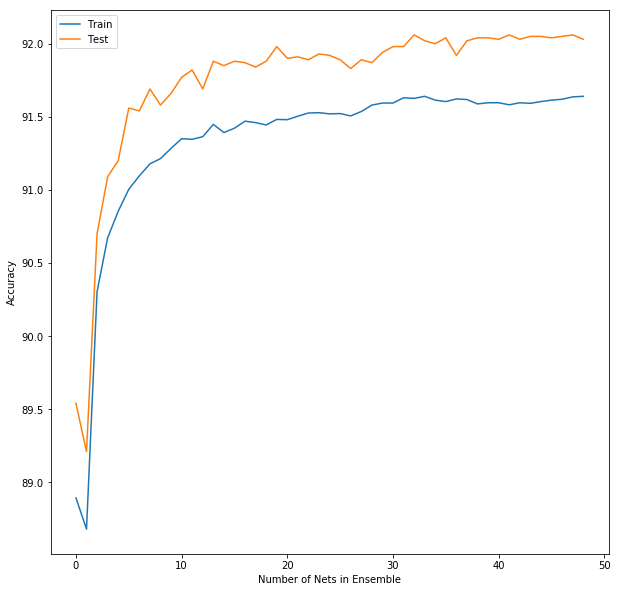# Ensembles of Convolutional Neural Networks¶

In :
import numpy as np
import scipy.stats as ss # for mode
import matplotlib.pyplot as plt
%matplotlib inline

import matplotlib.gridspec as gridspec
import pickle
import gzip

In :
import neuralnetworkConvolutionalClassifier as nn


Get data from DeepLearning Tutorial.

In :
with gzip.open('mnist.pkl.gz', 'rb') as f:
train_set, valid_set, test_set = pickle.load(f, encoding='latin1')

Xtrain = train_set
Ttrain = train_set.reshape((-1, 1))
Xtest = test_set
Ttest = test_set.reshape((-1, 1))

Xtrain.shape, Ttrain.shape, Xtest.shape, Ttest.shape

Out:
((50000, 784), (50000, 1), (10000, 784), (10000, 1))
In :
plt.figure(figsize=(10,10))
for i in range(100):
plt.subplot(10,10,i+1)
plt.imshow(-Xtrain[i,:].reshape((28,28)),interpolation='nearest',cmap='gray')
plt.axis('off')
plt.title(str(Ttrain[i]))
plt.tight_layout()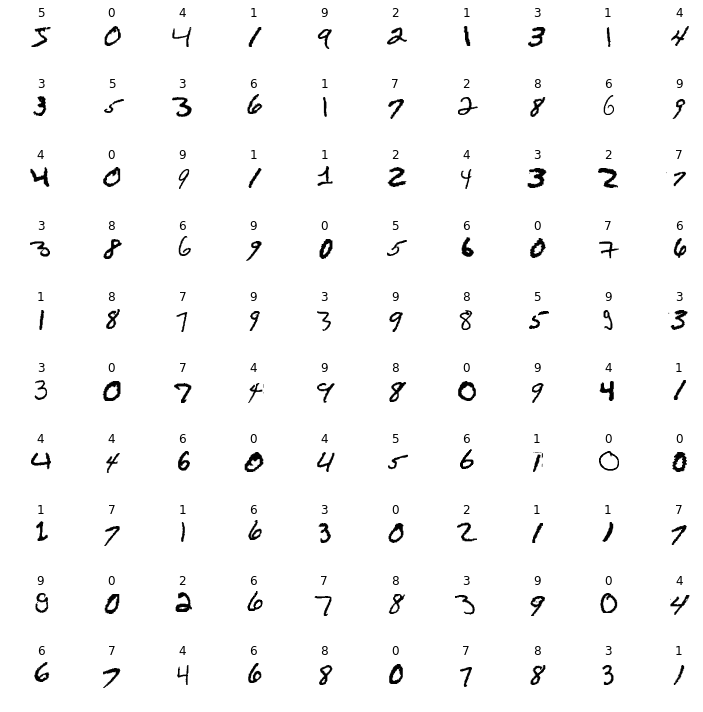We might run into memory issues, since our implementation of convolutional networks uses a lot of memory to create all of the small windows, or patches, of each image. Check out the python gc packages, discussed at this stackoverflow entry.

In :
import os
import psutil

def printMB():
process = psutil.Process(os.getpid())
print('{:.2f} MB'.format(process.memory_info().rss / 1024 / 1024))
printMB()

412.56 MB


Make a neural network with the first layer being a convolutional layer of 20 units, with each unit learning a 5x5 matrix of weights to be applied to all 5x5 patches in the image with a stride of 1. The second layer is a usual fully-connected layer with 50 units. The third and last layer is a multinomial output layer with 10 units, for the 10 classes.

In :
import gc

In :
gc.isenabled()

Out:
True

Try training with just 100 samples of each digit. A network will not do very well with such a small sample size.

In :
def percentCorrect(T, Y):
return np.mean(T == Y) * 100

In :
def trainEnsemble(Xtrain, Ttrain, nNetworks, nSamplesPerNetwork, nSCGIterations):
nSamples = Xtrain.shape
ensemble = []
for i in range(nNetworks):
indices = np.random.choice(range(nSamples),nSamplesPerNetwork)
Xsubset = Xtrain[indices,:]
Tsubset = Ttrain[indices,:]
nnet = nn.NeuralNetworkConvolutionalClassifier(1, [20, 50], 10,
inputShape=(28, 28), windowShape=(5, 5), windowStrides=(4, 4))
nnet.train(Xsubset, Tsubset, nSCGIterations)
# nnet.purge()
print('Net {:d} {:.2f}%'.format(i,percentCorrect(Tsubset, nnet.use(Xsubset))), end=', ')
ensemble.append(nnet)

return ensemble


In above code, the nnet.purge() function call that we discussed in class is not needed. The implementation in NeuralNetworkConvolutionalClassifier does not leave behind member variables that use a lot of memory, like self.Xw.

In :
nets = trainEnsemble(Xtrain, Ttrain, nNetworks=3, nSamplesPerNetwork=300, nSCGIterations=10)

Net 0 100.00%, Net 1 100.00%, Net 2 100.00%,

Running nnet.use on all test samples causes memory error, so let's apply it to batches of 5,000 samples. This works on my laptop.

In :
def predict(nets, X):
nNetworks = len(nets)
predictions = np.zeros((X.shape, nNetworks))
for i, net in enumerate(nets):
for first in range(0, X.shape, 5000):
predictions[first:first+5000, i:i+1] = net.use(X[first:first+5000, :])
# net.purge()
print('Net', i, 'done', end=', ')
return predictions

In :
def majorityVote(data, axis=1):
return ss.mode(data, axis=axis)

In :
ensemblePredictions = predict(nets, Xtest)
ensemblePrediction = majorityVote(ensemblePredictions)
print('\nEnsemble % Correct {:.2f}'.format(percentCorrect(Ttest, ensemblePrediction)))

Net 0 done, Net 1 done, Net 2 done,
Ensemble % Correct 86.61

In :
printMB()

528.23 MB

In :
print(gc.collect())
printMB()

141621
524.98 MB

In :
ensemblePredictions[:10,:]

Out:
array([[7., 7., 7.],
[2., 2., 2.],
[1., 1., 1.],
[0., 0., 0.],
[4., 4., 4.],
[1., 1., 1.],
[4., 4., 4.],
[7., 9., 9.],
[5., 5., 4.],
[9., 9., 7.]])
In :
plt.figure(figsize=(10,10))
for i in range(100):
plt.subplot(10,10,i+1)
plt.imshow(-Xtest[i,:].reshape((28,28)),interpolation='nearest',cmap='gray')
plt.axis('off')
plt.title(str(ensemblePredictions[i,:].astype(np.int)))
plt.tight_layout()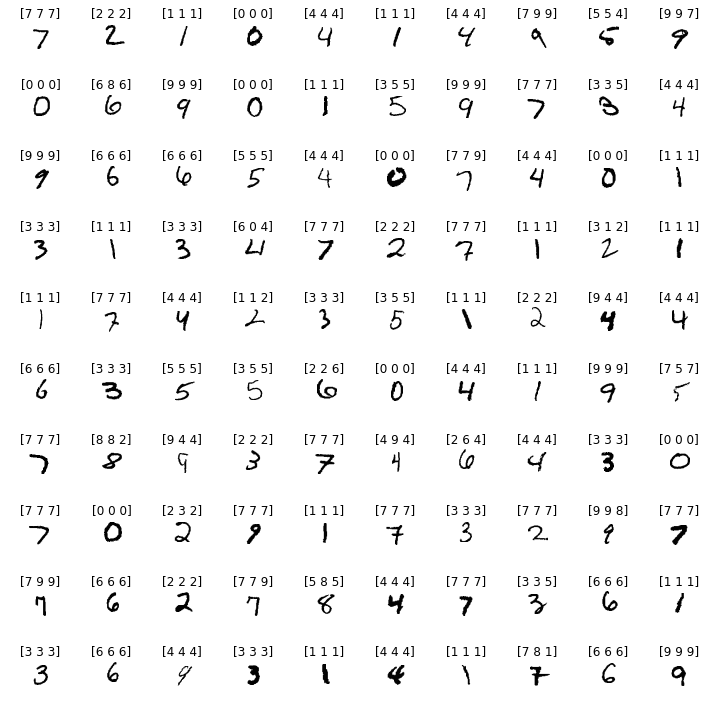In :
nets = trainEnsemble(Xtrain, Ttrain, nNetworks=10, nSamplesPerNetwork=1000, nSCGIterations=20)

Net 0 100.00%, Net 1 100.00%, Net 2 100.00%, Net 3 99.90%, Net 4 100.00%, Net 5 100.00%, Net 6 100.00%, Net 7 100.00%, Net 8 100.00%, Net 9 100.00%,
In :
predictionsByNetTrain = predict(nets, Xtrain)

Net 0 done, Net 1 done, Net 2 done, Net 3 done, Net 4 done, Net 5 done, Net 6 done, Net 7 done, Net 8 done, Net 9 done,
In :
plt.figure(figsize=(20, 10))
plt.plot(predictionsByNetTrain[:100,:],'bo')
plt.plot(np.vstack((range(100), range(100))), [*100, *100], 'y-');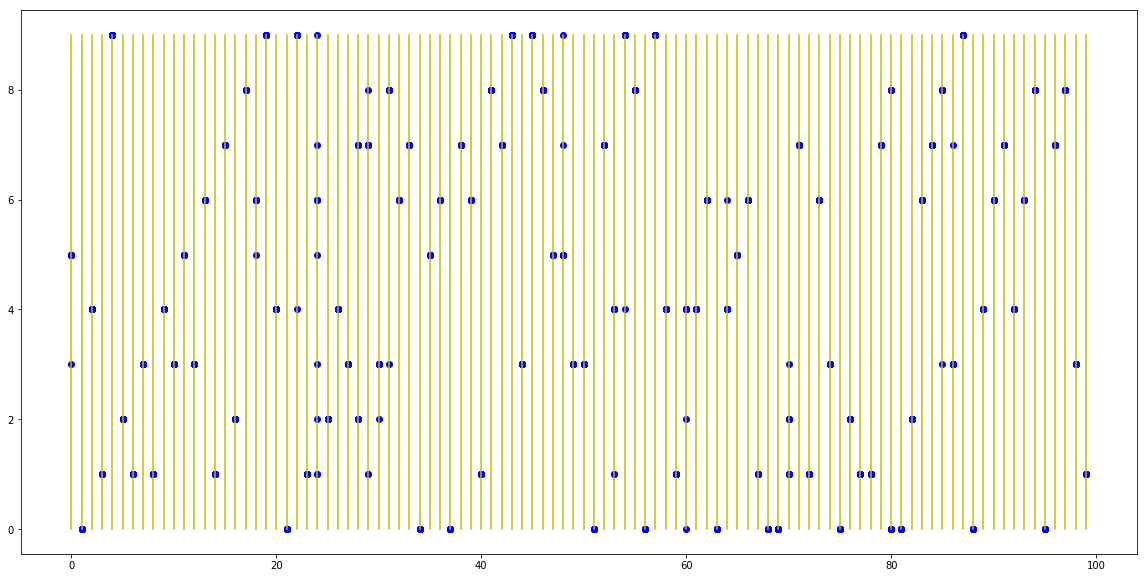In :
predictionsByNetTest = predict(nets, Xtest)

Net 0 done, Net 1 done, Net 2 done, Net 3 done, Net 4 done, Net 5 done, Net 6 done, Net 7 done, Net 8 done, Net 9 done,
In :
accuracies = []
for nNets in range(1, len(nets)):
ensemblePredictionTrain = majorityVote(predictionsByNetTrain[:, 0:nNets])
ensemblePredictionTest = majorityVote(predictionsByNetTest[:, 0:nNets])
accuracies.append([percentCorrect(Ttrain, ensemblePredictionTrain),
percentCorrect(Ttest, ensemblePredictionTest)] )

In :
plt.figure(figsize=(10, 10))
plt.plot(np.array(accuracies))
plt.legend(('Train','Test'))
plt.xlabel('Number of Nets in Ensemble')
plt.ylabel('Accuracy');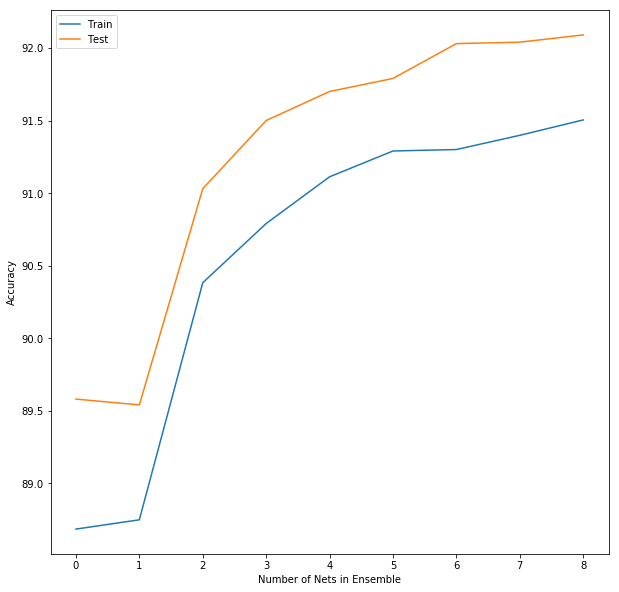In :
nets = trainEnsemble(Xtrain, Ttrain, nNetworks=50, nSamplesPerNetwork=1000, nSCGIterations=10)

Net 0 99.50%, Net 1 99.80%, Net 2 99.60%, Net 3 99.70%, Net 4 99.60%, Net 5 99.90%, Net 6 99.80%, Net 7 99.50%, Net 8 99.90%, Net 9 99.90%, Net 10 99.80%, Net 11 99.60%, Net 12 99.70%, Net 13 99.30%, Net 14 100.00%, Net 15 99.40%, Net 16 99.40%, Net 17 99.30%, Net 18 99.50%, Net 19 99.50%, Net 20 99.60%, Net 21 99.80%, Net 22 99.60%, Net 23 100.00%, Net 24 99.50%, Net 25 99.40%, Net 26 98.90%, Net 27 99.80%, Net 28 99.60%, Net 29 99.90%, Net 30 99.80%, Net 31 99.70%, Net 32 99.60%, Net 33 99.70%, Net 34 99.80%, Net 35 99.90%, Net 36 99.90%, Net 37 99.70%, Net 38 99.80%, Net 39 99.90%, Net 40 99.90%, Net 41 99.00%, Net 42 99.70%, Net 43 99.60%, Net 44 99.60%, Net 45 99.80%, Net 46 99.50%, Net 47 99.60%, Net 48 99.40%, Net 49 99.50%,
In :
print('nnet.use(Xtrain)...')
predictionsByNetTrain = predict(nets, Xtrain)
print('nnet.use(Xtest)...')
predictionsByNetTest = predict(nets, Xtest)

nnet.use(Xtrain)...
Net 0 done, Net 1 done, Net 2 done, Net 3 done, Net 4 done, Net 5 done, Net 6 done, Net 7 done, Net 8 done, Net 9 done, Net 10 done, Net 11 done, Net 12 done, Net 13 done, Net 14 done, Net 15 done, Net 16 done, Net 17 done, Net 18 done, Net 19 done, Net 20 done, Net 21 done, Net 22 done, Net 23 done, Net 24 done, Net 25 done, Net 26 done, Net 27 done, Net 28 done, Net 29 done, Net 30 done, Net 31 done, Net 32 done, Net 33 done, Net 34 done, Net 35 done, Net 36 done, Net 37 done, Net 38 done, Net 39 done, Net 40 done, Net 41 done, Net 42 done, Net 43 done, Net 44 done, Net 45 done, Net 46 done, Net 47 done, Net 48 done, Net 49 done, nnet.use(Xtest)...
Net 0 done, Net 1 done, Net 2 done, Net 3 done, Net 4 done, Net 5 done, Net 6 done, Net 7 done, Net 8 done, Net 9 done, Net 10 done, Net 11 done, Net 12 done, Net 13 done, Net 14 done, Net 15 done, Net 16 done, Net 17 done, Net 18 done, Net 19 done, Net 20 done, Net 21 done, Net 22 done, Net 23 done, Net 24 done, Net 25 done, Net 26 done, Net 27 done, Net 28 done, Net 29 done, Net 30 done, Net 31 done, Net 32 done, Net 33 done, Net 34 done, Net 35 done, Net 36 done, Net 37 done, Net 38 done, Net 39 done, Net 40 done, Net 41 done, Net 42 done, Net 43 done, Net 44 done, Net 45 done, Net 46 done, Net 47 done, Net 48 done, Net 49 done, 
In :
accuracies = []
for nNets in range(1, len(nets)):
ensemblePredictionTrain = majorityVote(predictionsByNetTrain[:, 0:nNets])
ensemblePredictionTest = majorityVote(predictionsByNetTest[:, 0:nNets])
accuracies.append([percentCorrect(Ttrain, ensemblePredictionTrain),
percentCorrect(Ttest, ensemblePredictionTest)] )
plt.figure(figsize=(10, 10))
plt.plot(np.array(accuracies))
plt.legend(('Train','Test'))
plt.xlabel('Number of Nets in Ensemble')
plt.ylabel('Accuracy');